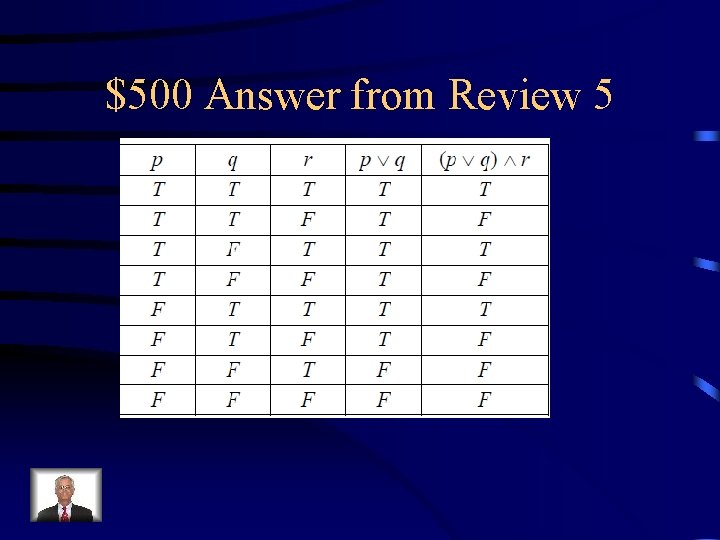# Jeopardy Review 1 Review 2 Review 3 Review

• Slides: 51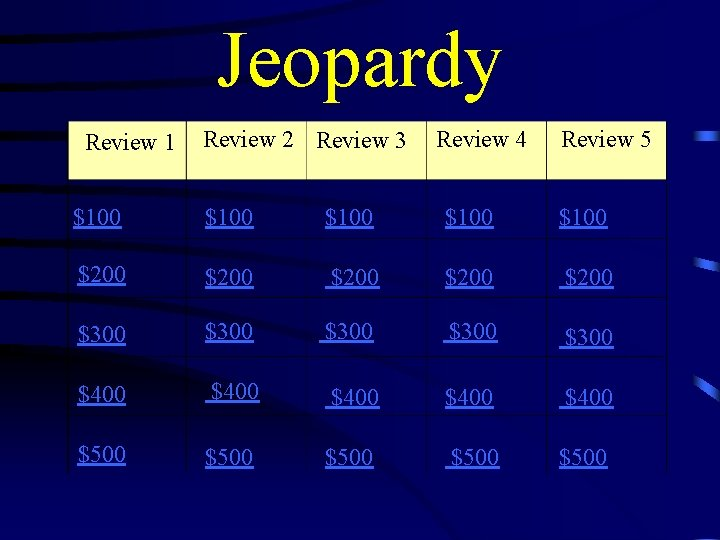Jeopardy Review 1 Review 2 Review 3 Review 4 Review 5 \$100 \$100 \$200 \$200 \$300 \$300 \$400 \$400 \$500 \$500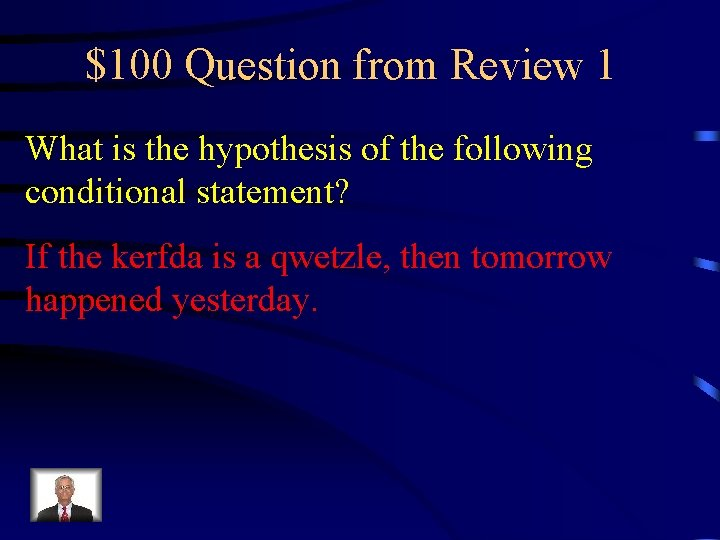\$100 Question from Review 1 What is the hypothesis of the following conditional statement? If the kerfda is a qwetzle, then tomorrow happened yesterday.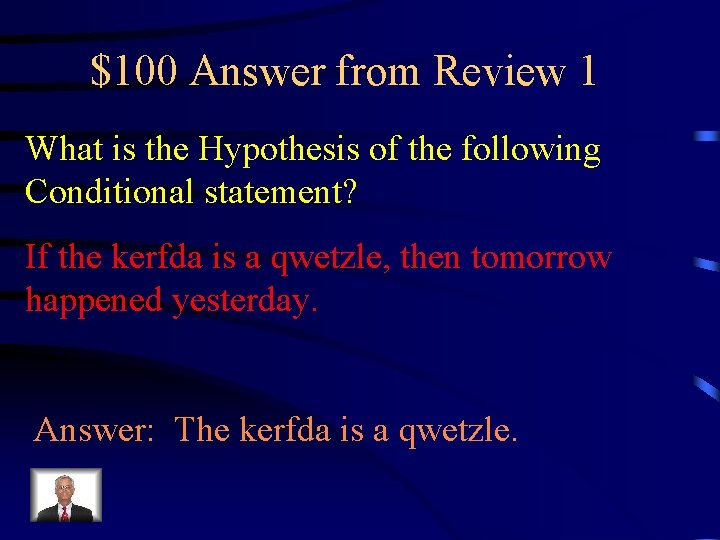\$100 Answer from Review 1 What is the Hypothesis of the following Conditional statement? If the kerfda is a qwetzle, then tomorrow happened yesterday. Answer: The kerfda is a qwetzle.\$200 Question from Review 1 What is the inverse of the following conditional statement? If I set my alarm, then I will be on time for work.\$200 Answer from Review 1 What is the inverse of the following conditional statement? If I set my alarm, then I will be on time for work. Answer: If I do not set my alarm, then I will not be on time for work.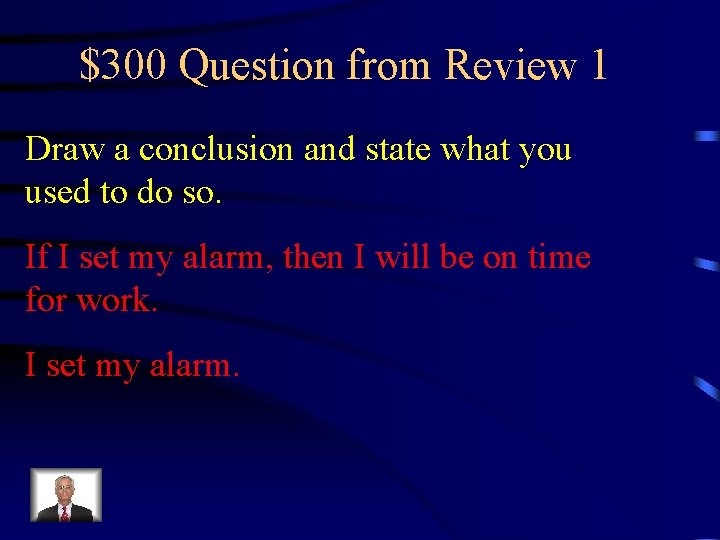\$300 Question from Review 1 Draw a conclusion and state what you used to do so. If I set my alarm, then I will be on time for work. I set my alarm.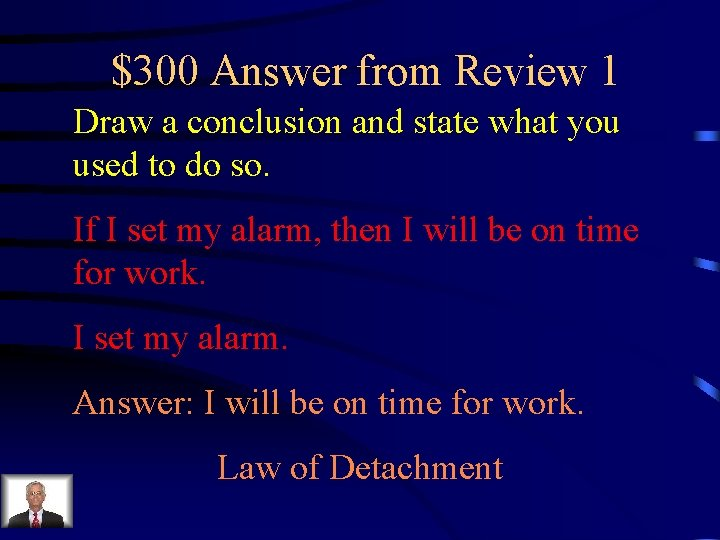\$300 Answer from Review 1 Draw a conclusion and state what you used to do so. If I set my alarm, then I will be on time for work. I set my alarm. Answer: I will be on time for work. Law of Detachment\$400 Question from Review 1 Give either the justification or statement for each step. Statements 1. 60 x -5(3 x + 2) = 15 x -7 2. 60 x -15 x -10 = 15 x -7 3. 45 x -10 = 15 x -7 4. 5. 30 x = 3 6. x = 1/10 Justifications 1. 2. 3. 4. Addition Property of Equality 5. 6.\$400 Answer from Review 1 Statements Justifications 1. 60 x -5(3 x + 2) = 15 x -7 2. 60 x -15 x -10 = 15 x -7 3. 45 x -10 = 15 x -7 4. 45 x = 15 x +3 5. 30 x = 3 6. x = 1/10 1. Given 2. Distributive Property 3. Adding like terms( something similar) 4. Addition Property of Equality 5. Subtraction Property of Equality 6. Division Property of Equality\$500 Question from Review 1\$500 Answer from Review 1 Statements Justifications 1. Given 2. Multiplication Property of Equality 3. Distributive Property 4. Addition Property of Equality 5. Division Property of Equality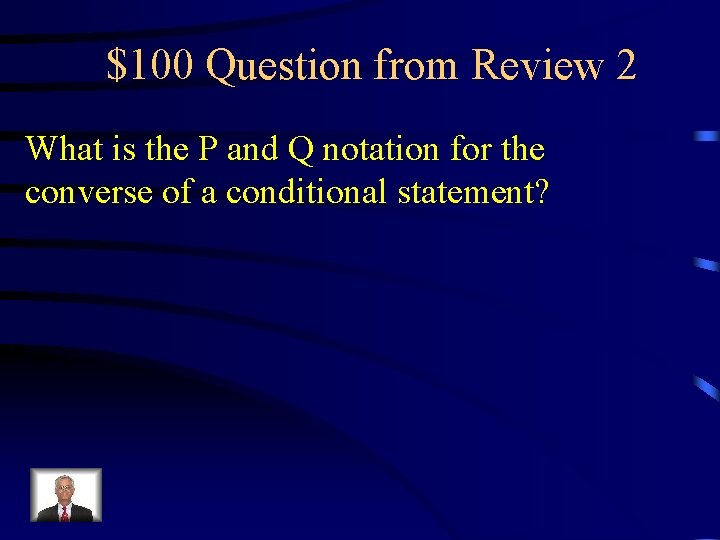\$100 Question from Review 2 What is the P and Q notation for the converse of a conditional statement?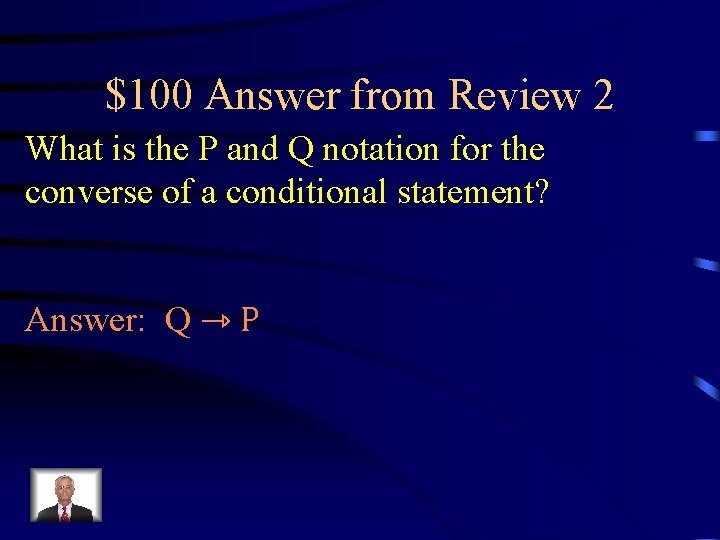\$100 Answer from Review 2 What is the P and Q notation for the converse of a conditional statement? Answer: Q ⇾ P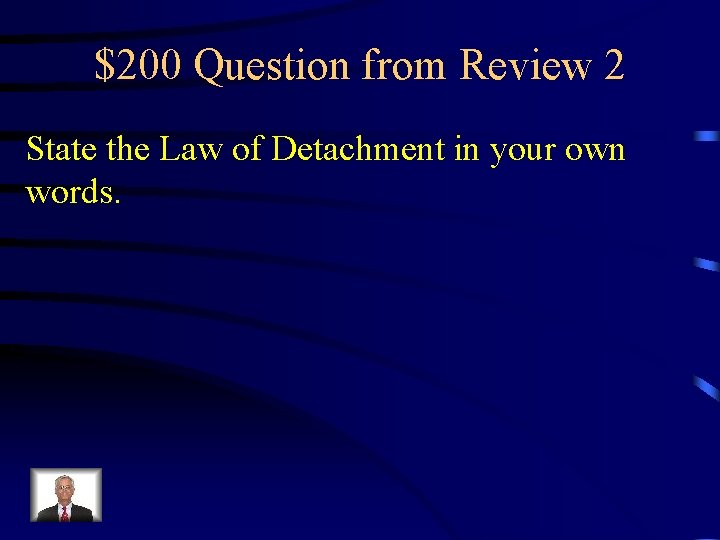\$200 Question from Review 2 State the Law of Detachment in your own words.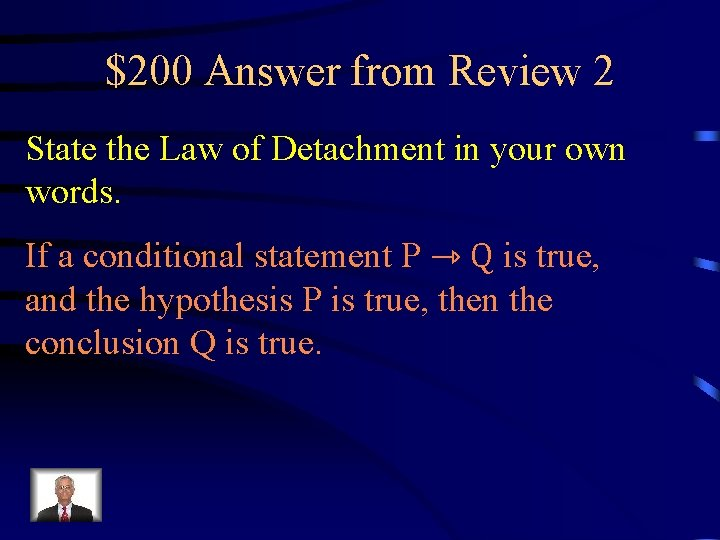\$200 Answer from Review 2 State the Law of Detachment in your own words. If a conditional statement P ⇾ Q is true, and the hypothesis P is true, then the conclusion Q is true.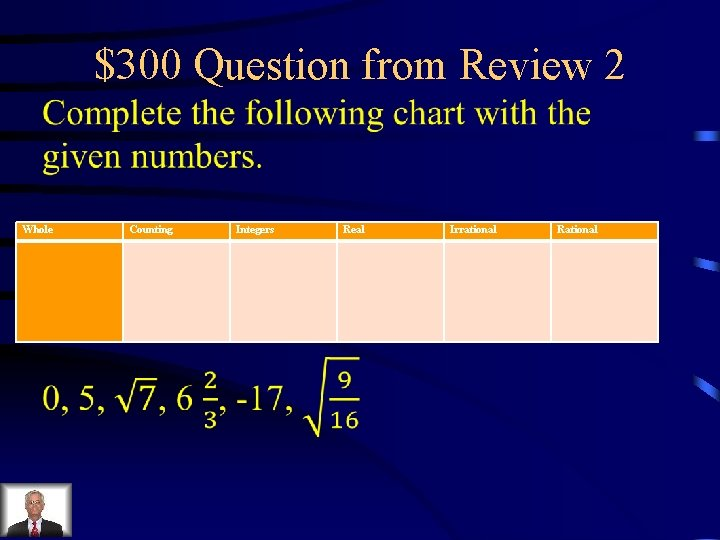\$300 Question from Review 2 Whole Counting Integers Real Irrational Rational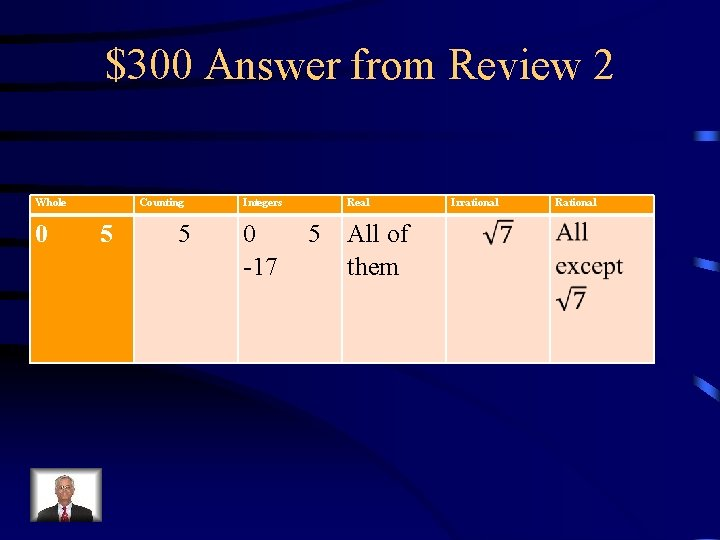\$300 Answer from Review 2 Whole Counting 0 5 5 Integers Real 0 5 All of -17 them Irrational Rational\$400 Question from Review 2 What conditional statement always has the same truth value as the contrapositive?\$400 Answer from Review 2 What conditional statement always has the same truth value as the contrapositive? Answer: The original conditional statement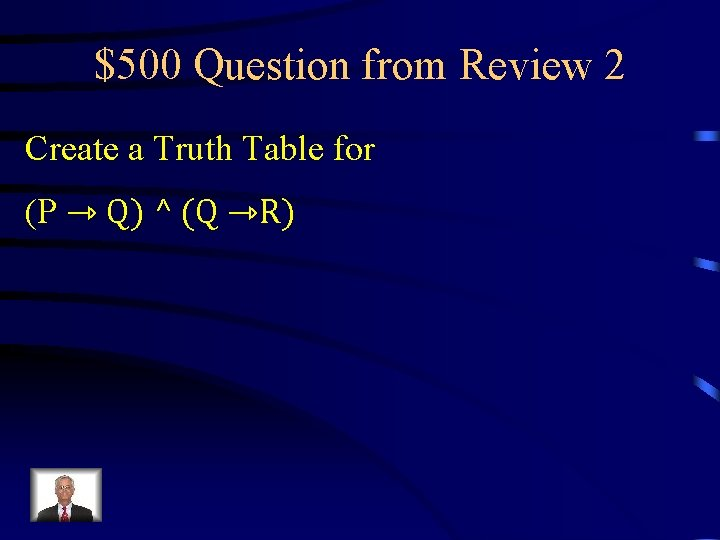\$500 Question from Review 2 Create a Truth Table for (P ⇾ Q) ^ (Q ⇾R)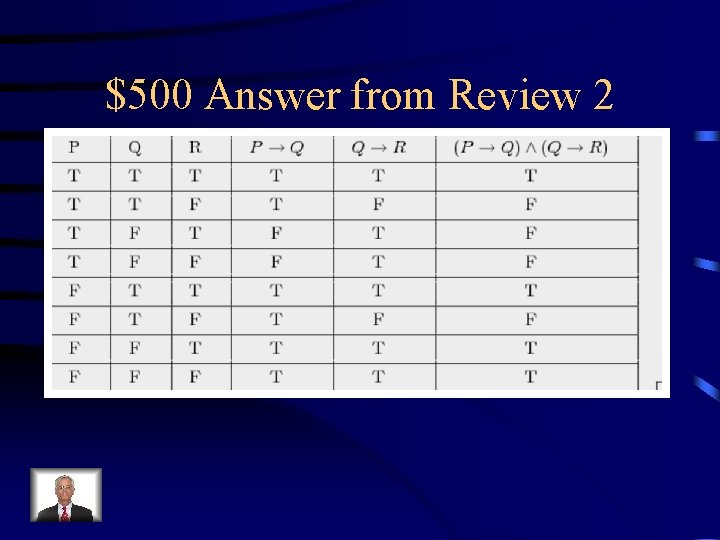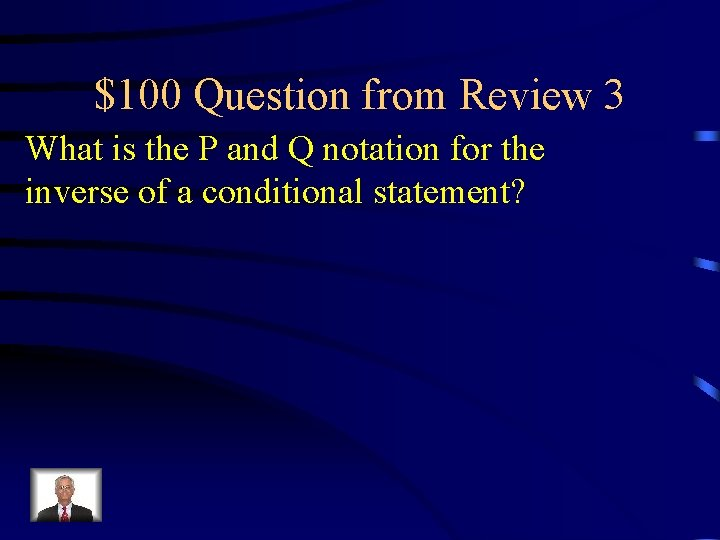\$100 Question from Review 3 What is the P and Q notation for the inverse of a conditional statement?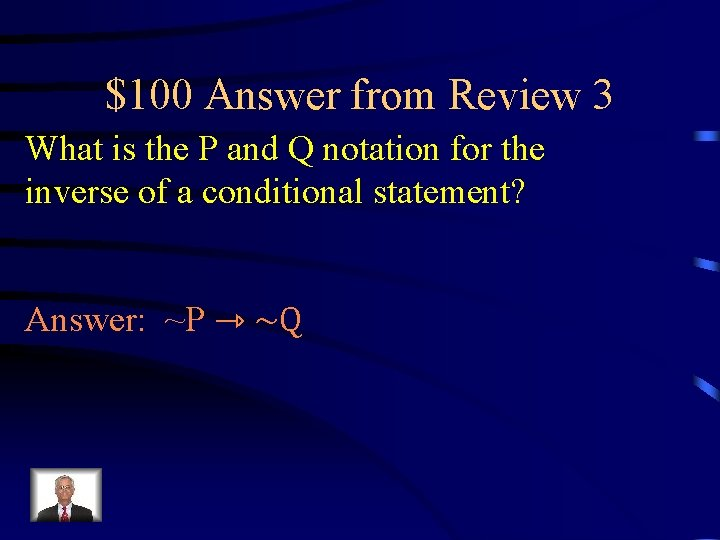\$100 Answer from Review 3 What is the P and Q notation for the inverse of a conditional statement? Answer: ~P ⇾ ~Q\$200 Question from Review 3 What is the contrapositive of the following conditional statement? If I get bored, then I will watch TV.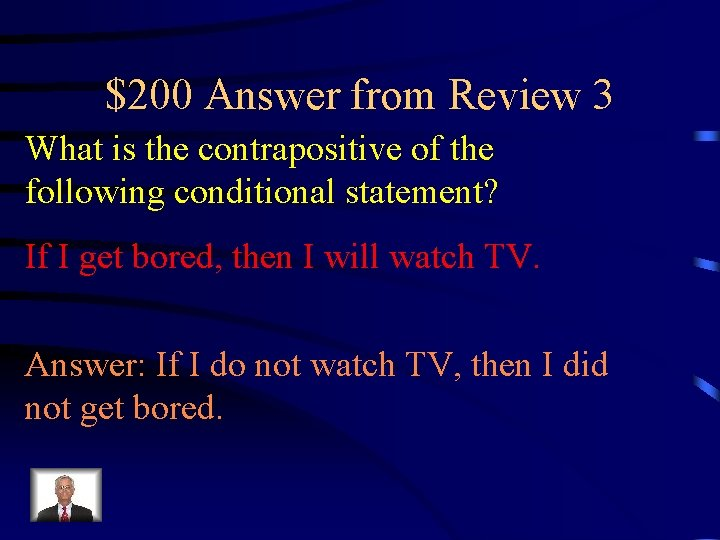\$200 Answer from Review 3 What is the contrapositive of the following conditional statement? If I get bored, then I will watch TV. Answer: If I do not watch TV, then I did not get bored.\$300 Question from Review 3 Draw a conclusion and state what you used to do so. If it rains, then Jimmy wont go to the park. If Jimmy doesn’t go to the park, then Jimmy will be sad.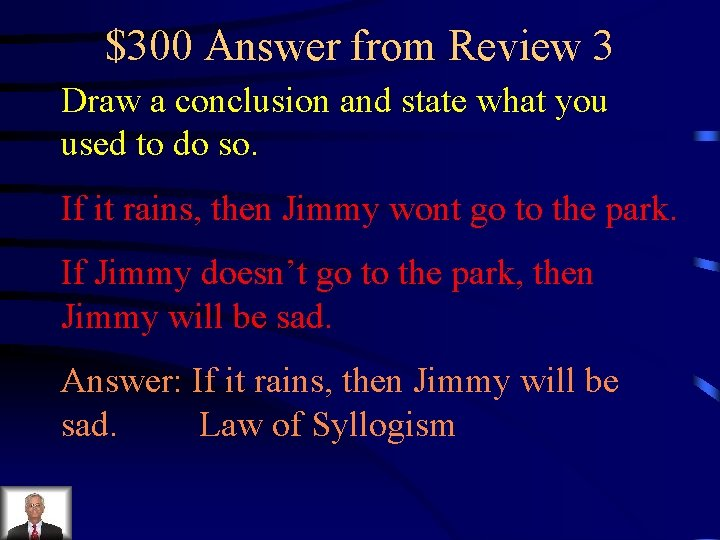\$300 Answer from Review 3 Draw a conclusion and state what you used to do so. If it rains, then Jimmy wont go to the park. If Jimmy doesn’t go to the park, then Jimmy will be sad. Answer: If it rains, then Jimmy will be sad. Law of Syllogism\$400 Question from Review 3 Create a Truth Table for (P ∧ Q) ⇾ (P ∨ Q)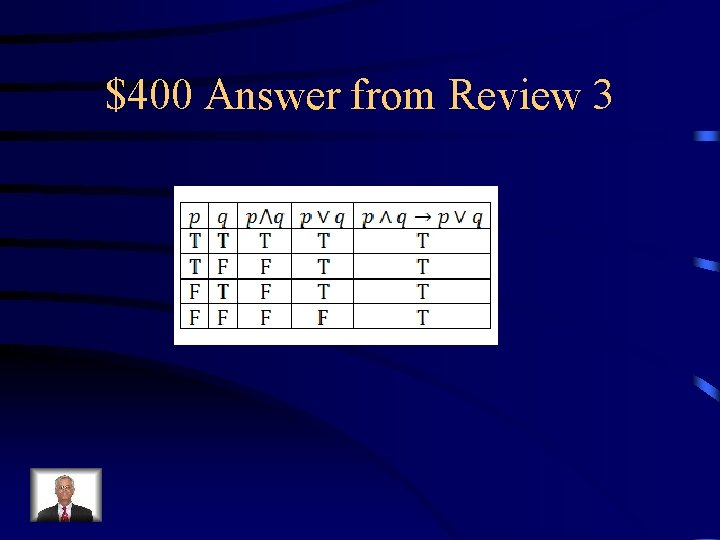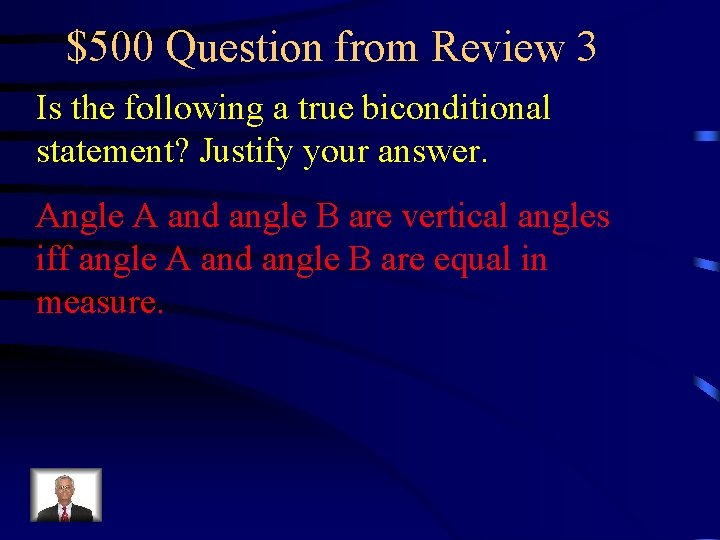\$500 Question from Review 3 Is the following a true biconditional statement? Justify your answer. Angle A and angle B are vertical angles iff angle A and angle B are equal in measure.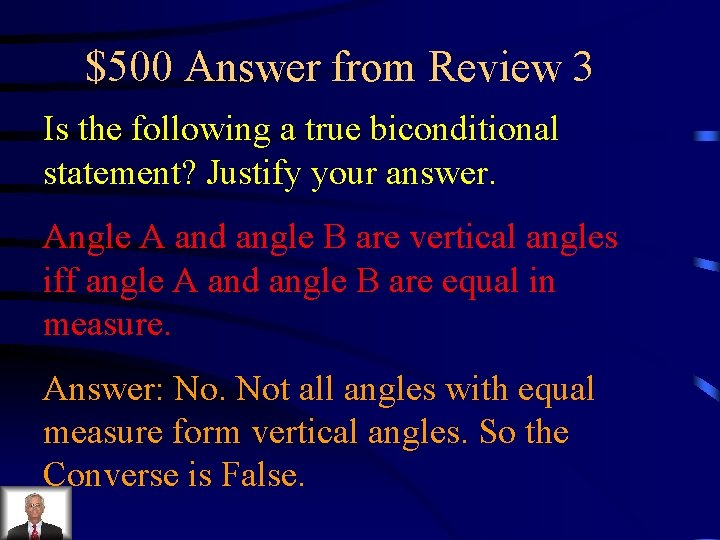\$500 Answer from Review 3 Is the following a true biconditional statement? Justify your answer. Angle A and angle B are vertical angles iff angle A and angle B are equal in measure. Answer: No. Not all angles with equal measure form vertical angles. So the Converse is False.\$100 Question from Review 4 What is the P and Q notation for the contrapositive of a conditional statement?\$100 Answer from Review 4 What is the P and Q notation for the contrapositive of a conditional statement? Answer: ~Q ⇾ ~P\$200 Question from Review 4 State the Law of Syllogism in your own words.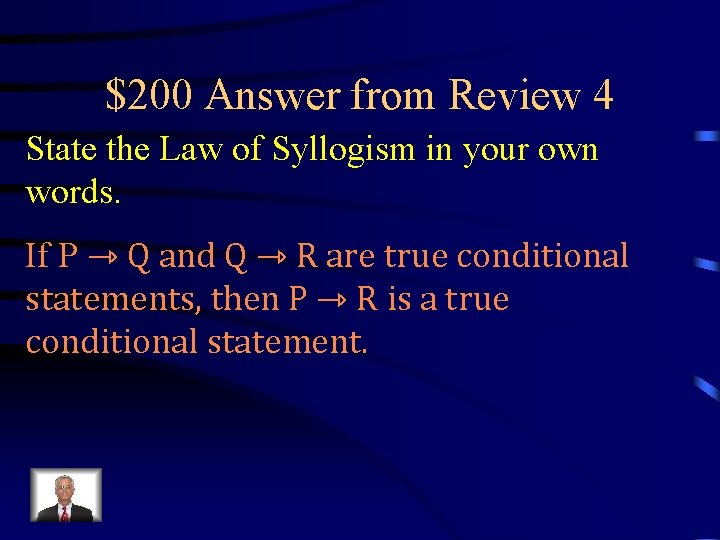\$200 Answer from Review 4 State the Law of Syllogism in your own words. If P ⇾ Q and Q ⇾ R are true conditional statements, then P ⇾ R is a true conditional statement.\$300 Question from Review 4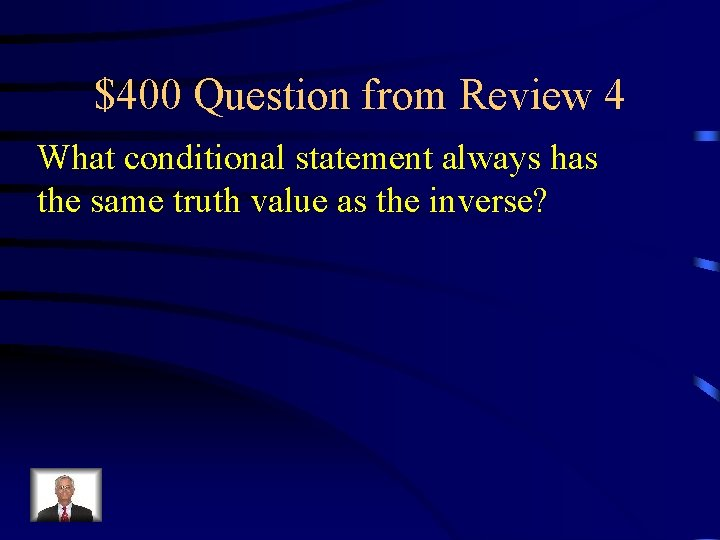\$400 Question from Review 4 What conditional statement always has the same truth value as the inverse?\$400 Answer from Review 4 What conditional statement always has the same truth value as the inverse? Answer: The converse.\$500 Question from Review 4 Is the following a true biconditional statement? Justify your answer. x = 3 Iff x 3 = 27.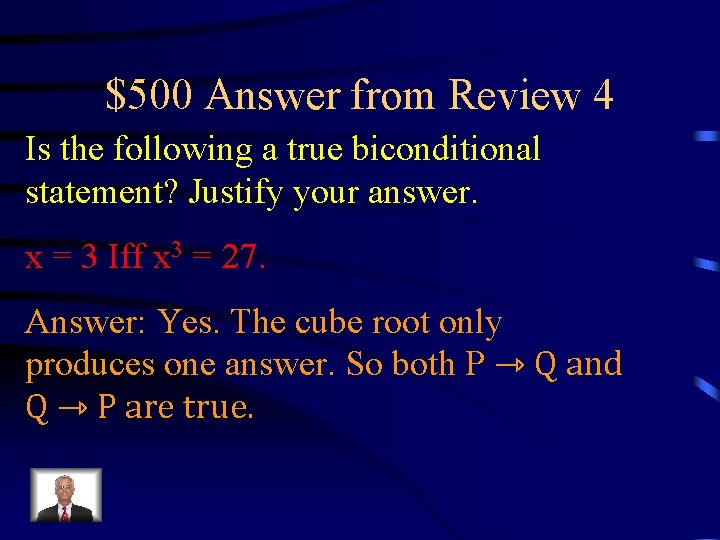\$500 Answer from Review 4 Is the following a true biconditional statement? Justify your answer. x = 3 Iff x 3 = 27. Answer: Yes. The cube root only produces one answer. So both P ⇾ Q and Q ⇾ P are true.\$100 Question from Review 5 What is the conclusion of the following conditional statement? If wizerdoodles are square, then spipdols will eat pizza.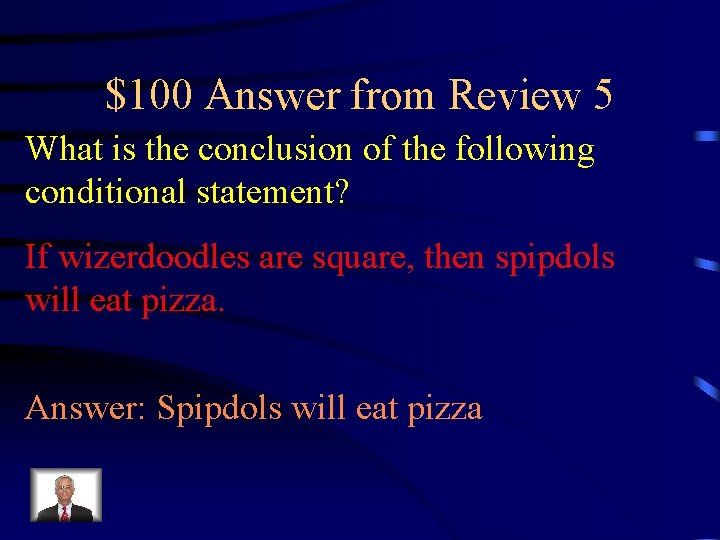\$100 Answer from Review 5 What is the conclusion of the following conditional statement? If wizerdoodles are square, then spipdols will eat pizza. Answer: Spipdols will eat pizza\$200 Question from Review 5 What is the converse of the following conditional statement? If I study for the test, then I will pass it.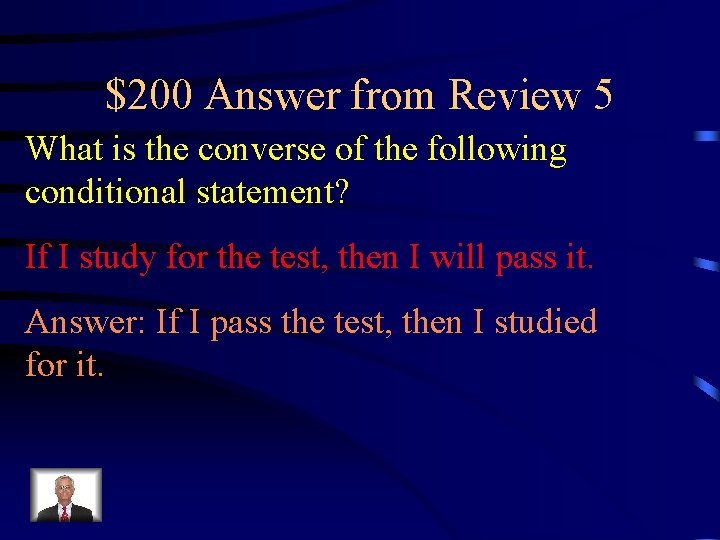\$200 Answer from Review 5 What is the converse of the following conditional statement? If I study for the test, then I will pass it. Answer: If I pass the test, then I studied for it.\$300 Question from Review 5 Find the next two objects in the pattern. What type of reasoning did you use?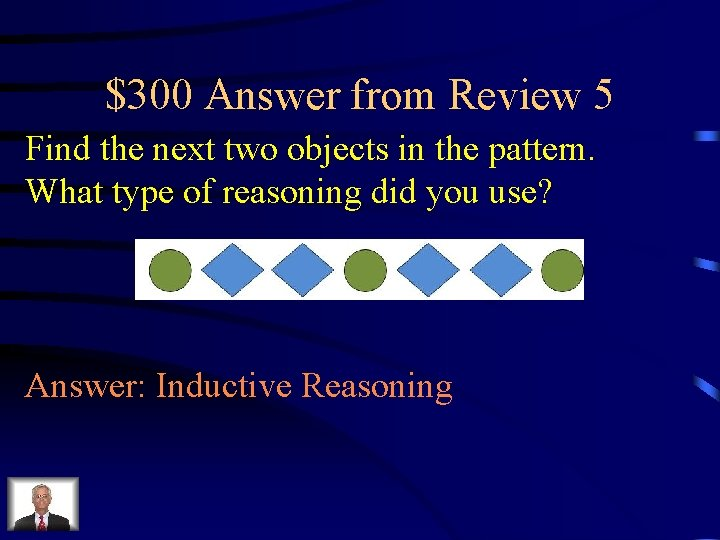\$300 Answer from Review 5 Find the next two objects in the pattern. What type of reasoning did you use? Answer: Inductive Reasoning\$400 Question from Review 5 Write the following as a biconditional statement. Bob and Mary will only go to the dance with each other.\$400 Answer from Review 5 Write the following as a biconditional statement. Bob and Mary will only go to the dance with each other. Answer: Bob and Mary will go to the dance iff they go together.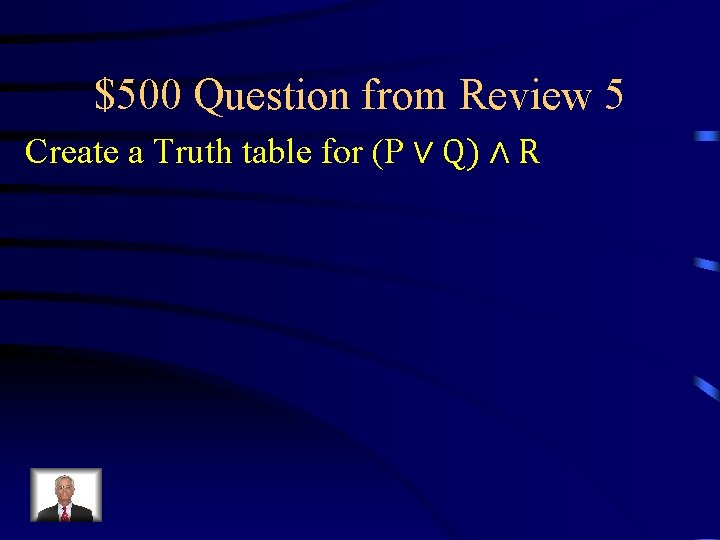\$500 Question from Review 5 Create a Truth table for (P ∨ Q) ∧ R